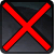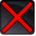# [juggernaut_flurry]Conditions

Used by

## Related effects, buffs and debuffs

• [0s] [not displayed]Effect #1

 Slot: Debuff Duration: 0s Tick rate: does not tick # occurrences: 0
• On Apply

Perform the following actions:

• Play appearance epp . flurry . npc . melee
• On Apply

Only when the following conditions are met:

• If difficulty is set to 16-man

Perform the following actions:

• Weapon Damage
- Is Special Ability = (bool) false
- Ignore Dual Wield Modifier = (bool) false
- Unknown (609) = (bool) false
- Flurry Blows Min = (int) 2
- Flurry Blows Max = (int) 2
- Level Cap = (int) 0
- Threat Percent = (float) 0
- Standard Health Percent Max = (float) 0.06
- Standard Health Percent Min = (float) 0.06
- Amount Modifier Fixed Max = (float) 0
- Amount Modifier Fixed Min = (float) 0
- Amount Modifier Percent = (float) -1
- Coefficient = (float) 0
- Health Steal Percentage = (float) 0
- Slots = Int(5)
• On Apply

Only when the following conditions are met:

• If difficulty is set to 8-man HM

Perform the following actions:

• Weapon Damage
- Is Special Ability = (bool) false
- Ignore Dual Wield Modifier = (bool) false
- Unknown (609) = (bool) false
- Flurry Blows Min = (int) 2
- Flurry Blows Max = (int) 2
- Level Cap = (int) 0
- Threat Percent = (float) 0
- Standard Health Percent Max = (float) 0.06
- Standard Health Percent Min = (float) 0.06
- Amount Modifier Fixed Max = (float) 0
- Amount Modifier Fixed Min = (float) 0
- Amount Modifier Percent = (float) -1
- Coefficient = (float) 0
- Health Steal Percentage = (float) 0
- Slots = Int(5)
• On Apply

Only when the following conditions are met:

• If difficulty is set to 8-man SM

Perform the following actions:

• Weapon Damage
- Is Special Ability = (bool) false
- Ignore Dual Wield Modifier = (bool) false
- Unknown (609) = (bool) false
- Flurry Blows Min = (int) 2
- Flurry Blows Max = (int) 2
- Level Cap = (int) 0
- Threat Percent = (float) 0
- Standard Health Percent Max = (float) 0.02
- Standard Health Percent Min = (float) 0.02
- Amount Modifier Fixed Max = (float) 0
- Amount Modifier Fixed Min = (float) 0
- Amount Modifier Percent = (float) -1
- Coefficient = (float) 0
- Health Steal Percentage = (float) 0
- Slots = Int(5)